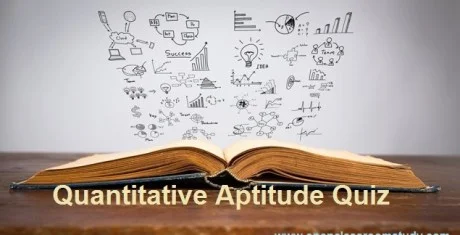New Student User - Use Code HELLO

# Mixed Aptitude Quiz - 8 : For IBPS PO Pre Exam 2016#### Ques 1.

Two cars of same length are running in in the same direction with speed 60 kmph and 90 kmph.The latter completely cross the man in 24sec. The length of the each bus is
Solution :
90 – 60 = 50
30×(5/18) = 25/3 m/s
Distance covered in 20sec = 24 ×(25/3) = 200
Length of each train = 200/2 = 100

#### Ques 2.

Two equal bottles are filled with water and milk. The amount of water in each container is 20% and 30%. What is the ratio of milk in both the bottles respectively ?

Solution :
1st Bottle Contains 80% of milk and 20% water
2nd  Bottle Contains 70% of milk and 30% water

Milk ratio = 80 : 70 = 8:7

#### Ques 3.

Ravi bought a car at 30% discount on its original price.He sold it with 50% increase on the price he bought it.The new sale price is by what percent more than the original price ?

Solution :
Let original price = Rs.100
30% discount = Rs.30
CP = 70
SP = (150/100)×70 = 105
Percentage = (105 – 100 )% = 5%

#### Ques 4.

Among a group of 5 men and 6 women , 4 members is to be selected for an event. Find the probability that at least one man is selected ?

Solution :
==>  1 – no boy is selected
==> 1 – 6c4/11c4 = 21/22

#### Ques 5.

Two pipes can fill the tank in 4hrs 5hrs respectively while the third pipe can empty the tank in 20hrs, if all the pipes are opened together, then the tank will be filled in

Solution :
1/4+1/5 -1/20
==>5+4-1/20
==> 8/20

20/8 => 2(1/2)hrs

#### Ques 6.

From the salary, Pavan spent 15% for house rent, 5% for children’s education and 15% for Entertainment. Now he left with Rs.26,000. His salary is

Solution :
10+15+10 = 35%
100-35 = 65% = 26,000

65% ------- 26,000

100% ------     ?

Salary = 40,000

#### Ques 7.

A and B invested in a business. They earned some profit which they divided in the ratio of 2:3. If P invested Rs.15,000, the amount invested by B is

Solution :
A : B = 2:3
==>15,000:B = 2:3
==> B = 22,500

#### Ques 8.

Milk and water are in the ratio of 3:2 in a mixture of 70 liters. How much water should be added so that the rate of milk and water become 2:3?

Solution :
5 units ----- 70
3 units ----- 42
Milk =42    water =28

==>42/(28+x) = 2/3
==> X = 35

#### Ques 9.

length of a rectangle is reduced by 40%. By what percent would the width have to be increased to maintain the original area?

Solution :
Width = 40*100/100-40
==> 4000/60 = 66.66%

#### Ques 10.

3 , 10 , 20 , 27 , 54 , 61 ,   ?

Solution :
3+7 = 10
10+10=20
20+7=27
27+27=54
54+7= 61
61+61 = 122